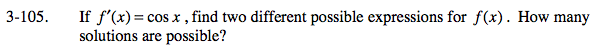### Home > CALC > Chapter 3 > Lesson 3.3.2 > Problem3-105

3-105.

If f ′(x) = cos x , find two different possible expressions for f(x). How many solutions are possible? Homework Help ✎What function has a derivative of cos(x)?

If you have not done so already, consider adding a constant to your first solution. Afterall, the derivative of a constant is 0.